# Pythagoras’ Theorem

Welcome to Class !!

In today’s Mathematics class, We will be discussing Pythagoras Theorem. We hope you enjoy the class!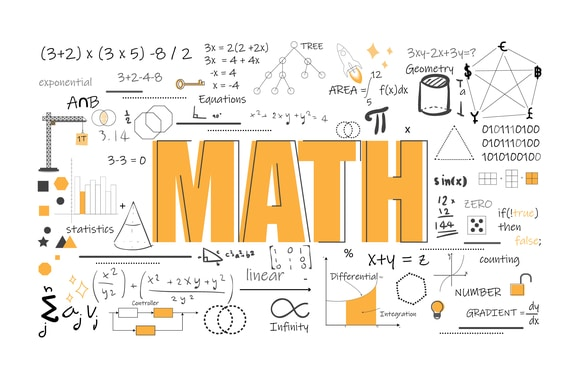CONTENT:

i. Pythagoras triple

ii  Pythagoras theorem

iii. Using Pythagoras theorem to solve other related problems.

PYTHAGORAS TRIPLE

The sides of a right-angled triangle can be related to the proof of Pythagoras Triple. A Pythagoras triple is a set of three whole numbers which gives lengths of the sides of a right-angled triangle.

Examples of some common Pythagoras triple are (3, 4, 5), (6, 8, 10). (5, 12, 13), etc.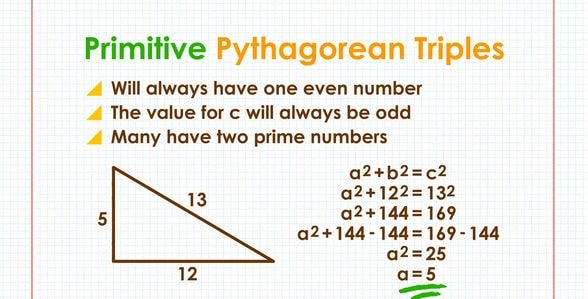Worked Example

Which of the following is a Pythagoras triple?

1. a) (15, 30, 35) b) (33, 56, 65)

Solution

152 + 302 = 225 + 900

= 1125

But 352 = 1225

(15, 30, 35) is not a Pythagoras triple

b) 332 + 562 = 1089 + 3136 = 4225

652 = 4225

Thus, 332 + 562 = 652

(33, 56, 65) is a Pythagoras triple.

Evaluation: Class Work

Find out which of the following are Pythagoras triples.

a) (12, 16, 20) b) (27, 36, 45) c) (14, 24, 28)

A) (12, 16, 20)

122 + 162 = 144 + 256 = 400

202 = 400

Thus, 122 + 162 = 20

(12, 16, 29) is a Pythagoras triple.

B) (27, 36, 45)

272 + 362 = 729 + 1296 = 2025

452 = 2025

Thus, 272 + 362 = 452

(27, 36, 45) is a Pythagoras triple

C) (14, 24, 28)

142 + 242 = 196 + 576 = 772

282  = 784

Thus, 14, 24, 28 is not a Pythagoras triple.

Reference: New General Mathematics Book 2, Chapter 7, Pages 150 – 151

Essential Mathematics for JSS Book 2, Chapter, 21, pages 218 and 219

### PYTHAGORAS THEOREMThe Pythagoras’ Theorem states that in any right-angled triangle, the square of the hypotenuse side is equal to the sum of the squares of the two sides.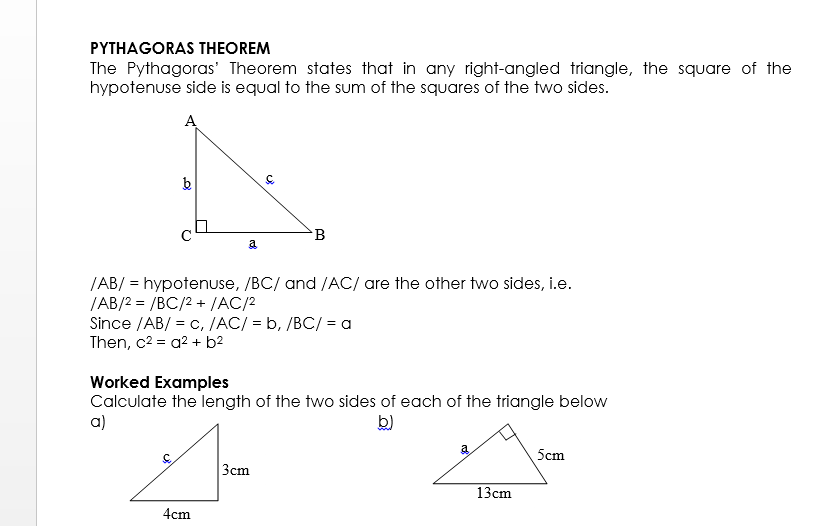A) Using Pythagoras rule

C2 = a2 + b2

a = 3, b = 4

c2 = 32 + 42 = 9 + 16

c2 = 25

c = 5m, the length of the third side is 5m.

B) Using Pythagoras rule

c2 = a2 + b2

C = 13, a = a b = 5

132 = a2 + 52

a2 = 169 – 25 = 144

a =

a = 12cm

Evaluations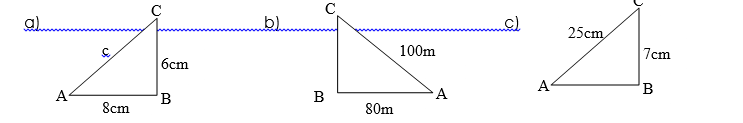a) /AC/2 = /AB/2 + /BC/2

AC = ?,          AB = 8cm,     BC = 6cm

AC2 = 82 + 62

AC =   = 10cm

b) /AC/2 = /AB/2– /BC/2

= 100m,        = 80m,     BC =?

1002 = 802 + /BC/2

1000 = 6400 + /BC/2

/BC/2 = 1000 – 6400

/BC/ =  = 60M

c) /AC/2 = /AB/2 + 72

AC = 25,        /AB/ = 72

252 = /AB/2 + 49

/AB/2 = 625 – 49 = 576

/AB/ =  = 24cm

Reference

NGM BK 2, chapter 17, pages 147 – 148

Essential mathematics for JSS BK 2, chapter 21, pages 215 – 218

###### USING PYTHAGORAS THEOREM TO SOLVE OTHER RELATED PROBLEM INVOLVING TRIANGLES

In some cases, we may have more than one right-angled triangle.

Worked examples

1. Calculate the length of the unknown in the following triangle: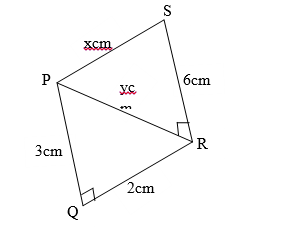Solution

A) PRS is a right-angled triangle, PQR is also a right-angled triangle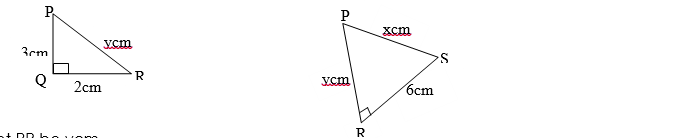Let PR be ycm

In triangle PQR; y2 = 32 + 22

= 9 + 4 = 13

y2 = 13

Let PS be xcm

In triangle PRS, x2 = y2 + 62

Substitute 13 for y2 in the formula

x2 = 13 + 62

x2 = 13 + 36

x2=  = 7

PS = 7cm

B) AD is the right-angled ABD. Let AB be ycm.

In triangle ABC, x2 = y2 + (8 + 12)2

Substitute 225 for y2 in the formula

X2 = 225 + 202

= 225 + 400 = 625

X =  = 25cm

When solving a triangle relating to decimal fraction and whole numbers, it is advisable to find the squares and square root from tables or multiplying the decimal by itself.

Evaluation

1. A ladder is 7.3m long and the foot of the ladder is 1.8m from the wall. How far up the wall is the ladder?
2. The distances between the opposite corner of a rectangular lawn is 30m, of the lawn, is 24m. Calculate the breadth of the lawn.

GENERAL EVALUATION

1. The distance between the opposite corners of a rectangular plot is 30m. The length of the plot is 24m. Calculate the breadth of the plot.
2. A student cycles from home to school, first eastwards to a road junction 12km from home, then southwards to school. If the school is 19km from home, how far is it from the road junction?

REVISION QUESTION:

1. A square top lid of a container has a diagonal 150cm. Find the length of one side of the lid.
2. ABCD is a rectangle. AB = xcm, BC = 9cm and the diagonal AC = 19cm. Calculate the value of x.

Essential Mathematics for JSS 2 Chapter 21 pages 268 – 271

Exercise 21.1 1a – b, 2a – d, 3a – b, page 270

WEEKEND ASSIGNMENT

1. The longest side of a right-angled triangle is called  A. hypotenuse    B. hypostasis    C. base    D. adjacent
2. Calculate the length of the diagonal of a 15m by 12m room.  A. 9m     B. 81m    C. 19m     D. 12m
3. Which of the following are Pythagorean triples?   A. 6, 8. 10      B. 12, 28, 32      C. 9, 12, 20     D. 13, 15, 17
4. Calculate the value of x in the diagram below.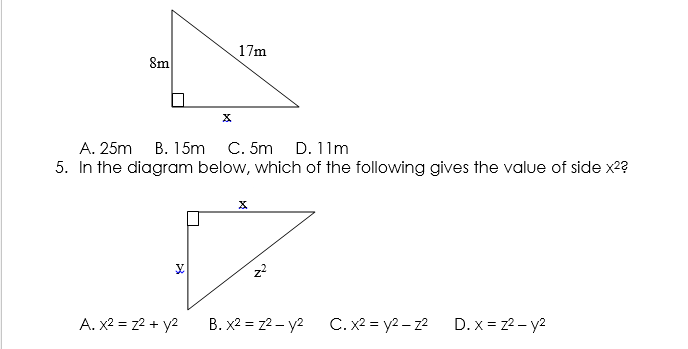THEORY

1. A flagpole 5m tall is supported by a wire that is fixed at point 3m from the base of the pole. Calculate to 1 d.p the length of the wire.
2. A square top lid of a container has a diagonal of 150cm. Find the length of one side of the lid.

We have come to the end of this class. We do hope you enjoyed the class?

Should you have any further question, feel free to ask in the comment section below and trust us to respond as soon as possible.

We have come to the end of this term and hence JSS2. It’s been a remarkable journey and we are glad that you have made it this far. For making it this far, we commend you for being resilient, you have taken charge of your education and future.

The Journey still continues though, we are moving on to JSS3. we hope to meet you there.

Are you a Parent? Share your quick opinion and win free 2-month Premium Subscription

### 1 thought on “ Pythagoras’ Theorem ”

1.Dibal Ibrahim John

excellent learning aid

Don`t copy text!